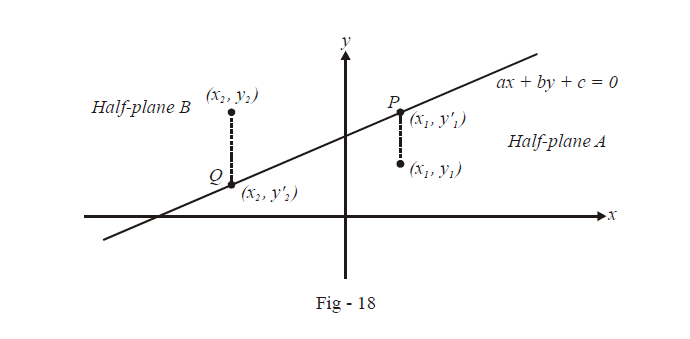# Half Planes Made by a Straight Line

Go back to  'Straight Lines'

$$\textbf{Art 6 : } \qquad\boxed{{\text{Half-planes}}}$$

Any straight line divides the Euclidean plane into two half planes. In this article, we wish to determine the half-plane in which an arbitrary point lies with respect to a given line.

Let the equation of the given line be  $$ax+by+c=0.$$ Consider two points $$\left( {{x}_{1}},{{y}_{1}} \right)\,\,\text{and}\,\,\left( {{x}_{2}},{{y}_{ 2}} \right)$$that lie in different half-planes with respect to this line:The point  $$\left( {{x}_{1}},{{y}_{1}} \right)$$ lies in the lower half-plane while $$\left( {{x}_{2}},{{y}_{2}} \right)$$ lies in the upper half plane. We require a condition on these co-ordinates which must be satisfied if the points lie in opposite half-planes. In Fig - 18, we have dropped verticle line segments from $$\left( {{x}_{1}},{{y}_{1}} \right)\,\,\text{and}\,\left( {{x}_{2}}{{y}_{2}} \right)$$ to the given line, intersecting it in P and Q respectively.

The co-ordinates of P and Q are $$({{x}_{1}},y_{1}^{'})$$ and  $$({{x}_{2}},y_{2}^{'})$$ respectively where $$y_{1}^{'}\ne {{y}_{1}}\,\,\text{and}\,\,y_{2}^{'}\ne {{y}_{2}}$$ .

Since P, Q lie on the given line, their co-ordinates must satisfy the equation of the line. Thus,

\begin{align}& \qquad\;\; a{{x}_{1}}+by_{1}^{\mathbf{'}}+c=0\qquad \Rightarrow \qquad y_{1}^{\mathbf{'}}=-\frac{\left( a{{x}_{1}}+c \right)}{b} \\ & and\quad a{{x}_{2}}+by_{2}^{\mathbf{'}}+c=0\qquad \Rightarrow \qquad y_{2}^{\mathbf{'}}=-\frac{\left( a{{x}_{2}}+c \right)}{b} \end{align}

Now, from Fig - 18 we have

\begin{align}&\qquad \qquad \qquad\quad\; {y_1} < y_1^{\mathbf{'}}\quad\text{and}\quad{y_2} > y_2^{\mathbf{'}} \\& \Rightarrow \;\;\quad {y_1} < - \frac{{\left( {a{x_1} + c} \right)}}{b}\quad \text{and} \quad{y_2} > - \frac{{\left( {a{x_2} + c} \right)}}{b} \\& \Rightarrow \quad \frac{{a{x_1} + b{y_1} + c}}{b} < 0\quad \text{and} \quad\frac{{a{x_2} + b{y_2} + c}}{b} > 0 \\& \Rightarrow \qquad\quad \; a{x_1} + b{y_1} + c\quad \text{and} \quad a{x_2} + b{y_2} + c\,\,\,{\text{are of opposite signs}}{\text{.}} \\ \end{align}

This is the required condition. Translated into words, it says that for two points lying in opposite half-planes, their co-ordinates when substituted respectively into the equation of the line must give expressions of opposite signs. (For two points in the same half-plane, the signs would be the same).

As a corollary, observe that a point (x1, y1) lies in the same half–plane or opposite half–plane in which the origin lies accordingly as $$\left( {a{x_1} + b{y_1} + c} \right)$$ and c are of the same sign or opposite signs respectively.